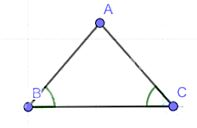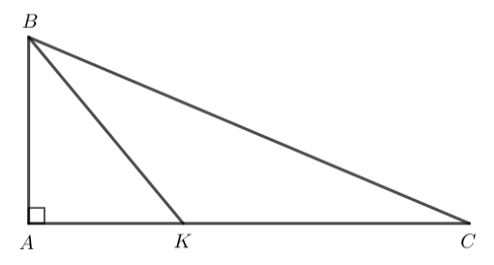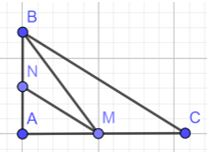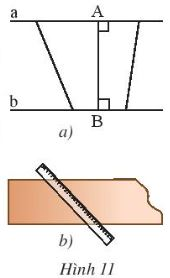## Solve Exercises Lesson 4: Perpendicular and oblique lines (C8 Math 7 Horizon) – Math Book

Solution for Exercise 4: Perpendicular and oblique lines (C8 Math 7 Horizons)
==========

### Solution 1 page 66 Math textbook 7 Creative horizon volume 2 – CTST

a) Compare the angles of triangle ABC with AB = 4 cm, BC = 7 cm, AC = 6 cm.

b) Compare the sides of triangle ABC with \(\widehat A\)\( = {50^o}\),\(\widehat C\)\( = {50^o}\)

Detailed instructions for solving Lesson 1

Solution method

– Use the theorem about the angle opposite the side in a triangle

– Use the isosceles triangle property and calculate the remaining angle of the triangle to compare the lengths of the sides

Detailed explanation

a) According to the problem, we have AB = 4cm, BC = 7cm, AC = 6cm

There is angle opposite side AB is angle C, angle A is opposite side BC, angle B is opposite side AC

According to the theorem about the angle opposite the larger side, we have:

\( \Rightarrow \widehat A > \widehat B > \widehat C\)

b)Since \(\widehat{B}=\widehat{C}\) then triangle ABC is isosceles at A

\( \Rightarrow AB = AC\)

Applying the sum of three angles theorem in triangle ABC, there are:

\( \Rightarrow \widehat A = {180^o} – {100^0} = {80^o}\)

\( \Rightarrow \widehat A > \widehat B;\widehat C\)

\( \Rightarrow BC\) is the largest side of triangle ABC

According to the theorem of the angle opposite the larger side, the greater is

–>

— *****

### Solution 2 page 66 Math textbook 7 Creative horizon volume 2 – CTST

Let ABC be a triangle with \(\widehat A = \)\({100^0}\),\(\widehat B\)\( = {40^o}\).

a) Find the largest side of triangle ABC.

b) What is triangle ABC? Why?

Detailed instructions for solving Lesson 2

Solution method

– Use the theorem about the angle opposite the side in a triangle

– Use isosceles triangle property

Detailed explanation

a) According to the summation theorem of 3 angles in a triangle, we have:

\( \Rightarrow \widehat A + \widehat B + \widehat C = {180^o}\)

\( \Rightarrow \widehat C = {180^o} – {100^o} – {40^o} = {40^o}\)

\( \Rightarrow \widehat A > \widehat C\) and \(\widehat A > \widehat B\)

\( \Rightarrow \) BC is the largest side of triangle ABC since it is opposite angle A

b) Consider triangle ABC with:

\(\widehat C = \widehat B = {40^o}\)( prove a )

\( \Rightarrow \) ABC is an isosceles triangle

–>

— *****

### Solve problem 3 page 66 Math textbook 7 Creative horizon volume 2 – CTST

Let ABC be a right triangle at A with \(\widehat B > {45^o}\)

a) Compare the sides of the triangle

b) Take any point K on the line segment AC. Compare the lengths of BK and BC.

Detailed instructions for solving Lesson 3

Solution method

Use the theorem about the angle opposite the side in a triangle

Detailed explanationa) Since triangle ABC is right-angled at A, \(\widehat{A}=90^0; \widehat{B}+\widehat{C}=90^0\)

Because \(\widehat B > {45^o} \Rightarrow \widehat C < {45^o} \Rightarrow \widehat A > \widehat B > \widehat C \Rightarrow BC > AC > AB\)

b) Since \(\widehat {BKC}\) is the exterior angle at the vertex K of triangle ABK, \(\widehat {BKC}>\(\widehat {BAK}=90^0\)

Considering triangle BCK, we have:

\(\widehat {BKC} > {90^o} > \widehat {BCK}\)

\( \Rightarrow BC > BK\) (the relationship between the angle and the opposite side in a triangle)

–>

— *****

### Solution 4 page 66 Math textbook 7 Creative horizon volume 2 – CTST

Observe Figure 10.

a) Find the shortest of the segments BA, BM, BC.

b) Find the shortest in the segments MA, MN, MB.

c) Prove that MA < BC.Detailed instructions for solving Lesson 4

Solution method

– Based on the properties from a point outside the line, the perpendicular is shorter than the oblique lines.

– We prove MA < AC < BC

Detailed explanation

a) BA is a perpendicular;

BM and BC are diagonal lines from B to line AC

We get the shortest path is the perpendicular, so BA is the shortest.

b) Similar to sentence a

MA is the perpendicular;

MN and MB are diagonal lines from M to line AB

We get the shortest path is the perpendicular, so MA is the shortest.

c) Consider triangle ABC, right angled at A

\( \Rightarrow \widehat A = {90^o}\)\( \Rightarrow \)A is the largest angle of triangle ABC

\( \Rightarrow \) BC > AC (opposite and side theorem)

Since M is in the middle of AC, AM < AC

\( \Rightarrow \) AM < AC < BC

So AM < BC

–>

— *****

### Solve problem 5 page 66 Math textbook 7 Creative horizon volume 2 – CTST

In Figure 11a, we call the length of line segment AB the distance between two parallel lines a and b.

a) A wooden brace with two parallel sides (Figure 11b). The width of the wooden brace is the distance between those two edges. Indicate whether the width of the wooden brace is the shortest distance from a point on one edge to a point on the other edge.

b) To measure the width of the splint, how should we set the ruler? Why?Detailed instructions for solving Lesson 5

Solution method

Use the relationship between perpendicular and oblique lines.

Detailed explanation

a) The width of the wooden brace is the shortest distance from a point on one side to a point on the other side (the perpendicular segment is the shortest).

b) We put the ruler perpendicular to the two edges of the brace because the width of the brace is the distance between the two edges of the brace

–>

— *****